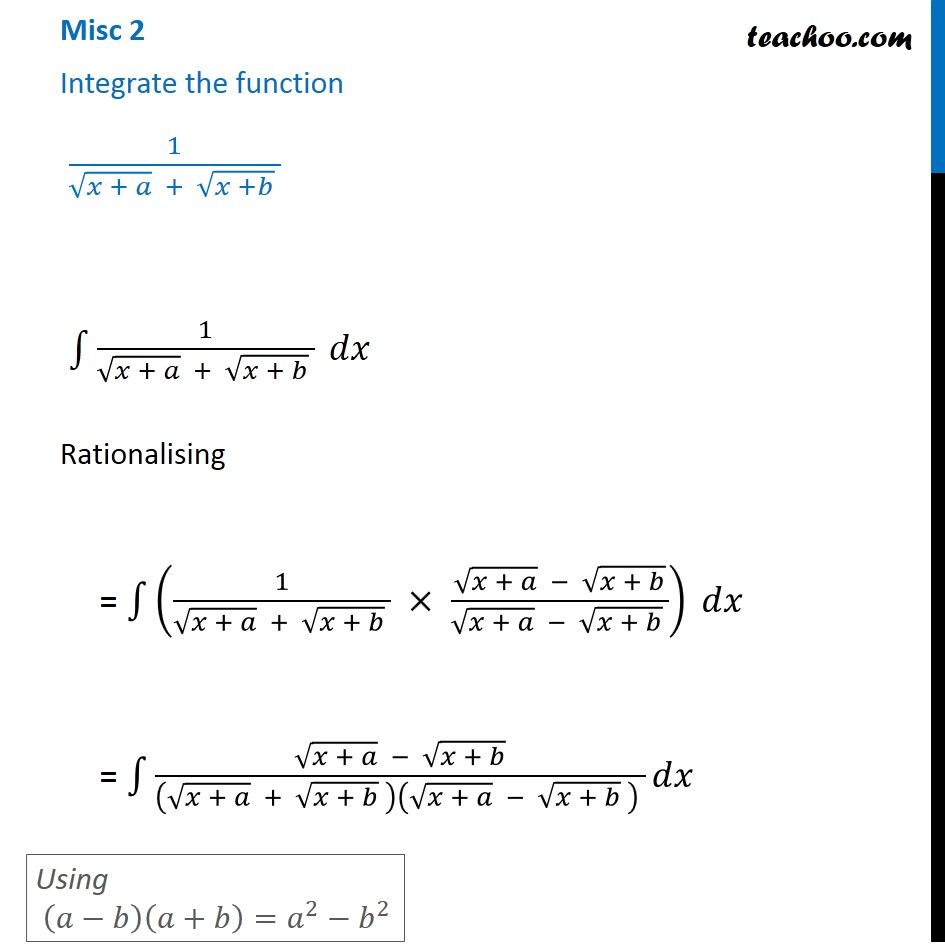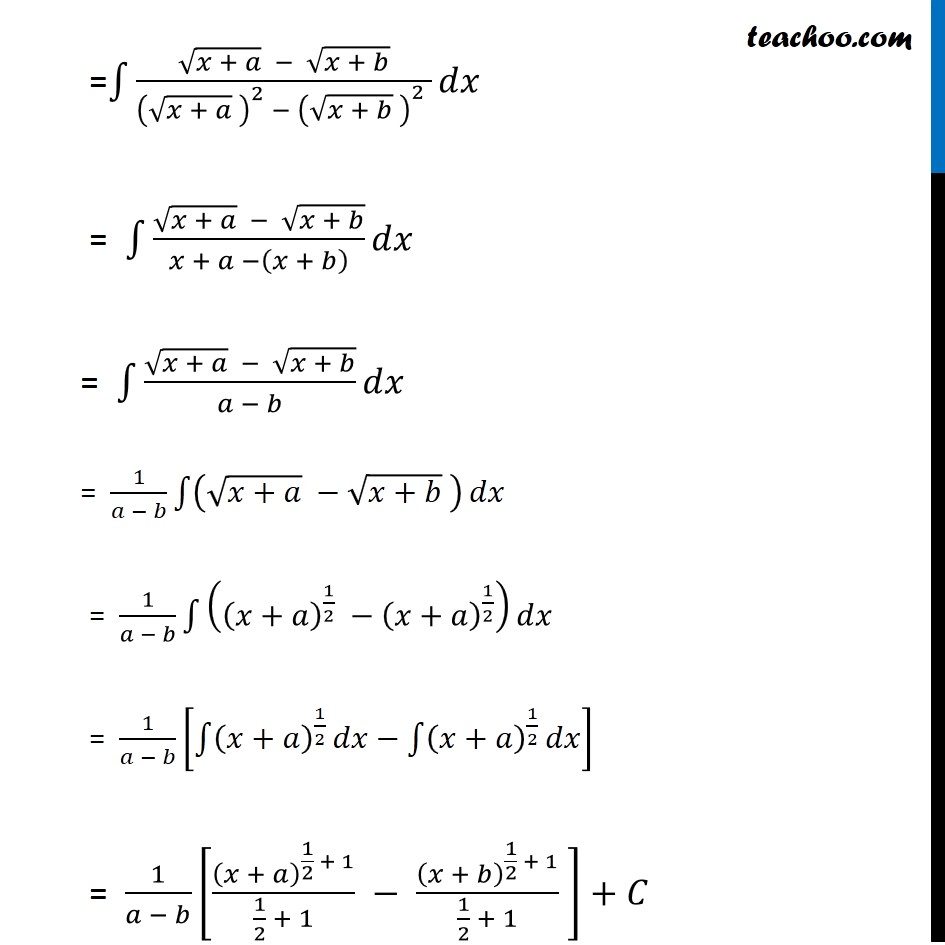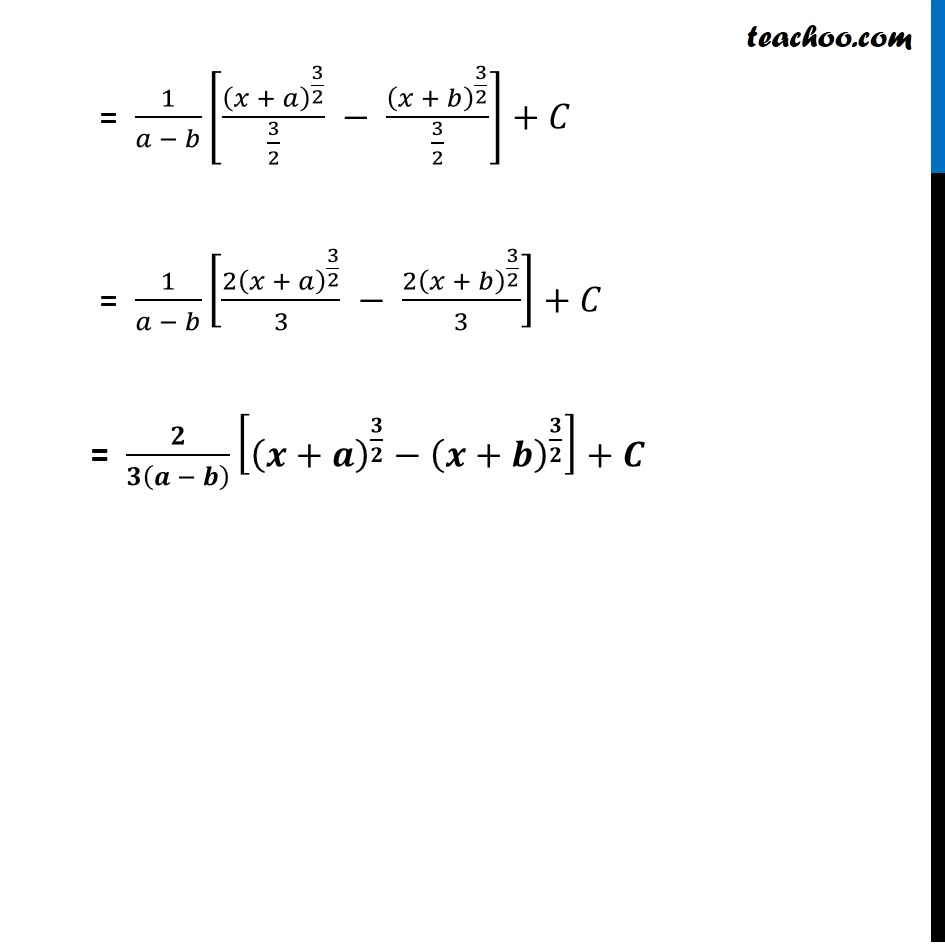Miscellaneous

Chapter 7 Class 12 Integrals
Serial order wiseLearn in your speed, with individual attention - Teachoo Maths 1-on-1 Class

### Transcript

Misc 2 Integrate the function 1/(√(𝑥 + 𝑎) + √(𝑥 +𝑏) ) ∫1▒〖1/(√(𝑥 + 𝑎) + √(𝑥 + 𝑏) ) 𝑑𝑥〗 Rationalising = ∫1▒〖(1/(√(𝑥 + 𝑎) + √(𝑥 + 𝑏) ) × (√(𝑥 + 𝑎) − √(𝑥 + 𝑏))/(√(𝑥 + 𝑎) − √(𝑥 + 𝑏) )) 𝑑𝑥〗 = ∫1▒〖(√(𝑥 + 𝑎) − √(𝑥 + 𝑏))/((√(𝑥 + 𝑎) + √(𝑥 + 𝑏) )(√(𝑥 + 𝑎) − √(𝑥 + 𝑏) ) ) 𝑑𝑥〗 Using (𝑎−𝑏)(𝑎+𝑏)=𝑎^2−𝑏^2 =∫1▒〖(√(𝑥 + 𝑎) − √(𝑥 + 𝑏))/((√(𝑥 + 𝑎) )^2 − (√(𝑥 + 𝑏) )^2 ) 𝑑𝑥〗 = ∫1▒〖(√(𝑥 + 𝑎) − √(𝑥 + 𝑏))/(𝑥 + 𝑎 −(𝑥 + 𝑏) ) 𝑑𝑥〗 = ∫1▒〖(√(𝑥 + 𝑎) − √(𝑥 + 𝑏))/(𝑎 − 𝑏) 𝑑𝑥〗 = 1/(𝑎 − 𝑏) ∫1▒(√(𝑥+𝑎) −√(𝑥+𝑏) ) 𝑑𝑥 = 1/(𝑎 − 𝑏) ∫1▒((𝑥+𝑎)^(1/2) −(𝑥+𝑎)^(1/2) ) 𝑑𝑥 = 1/(𝑎 − 𝑏) [∫1▒(𝑥+𝑎)^(1/2) 𝑑𝑥−∫1▒(𝑥+𝑎)^(1/2) 𝑑𝑥] = 1/(𝑎 − 𝑏) [(𝑥 + 𝑎)^(1/2 + 1)/(1/2 + 1) − (𝑥 + 𝑏)^(1/2 + 1)/(1/2 + 1) ] + 𝐶 = 1/(𝑎 − 𝑏) [(𝑥 + 𝑎)^(3/2)/(3/2) − (𝑥 + 𝑏)^(3/2)/(3/2)] + 𝐶 = 1/(𝑎 − 𝑏) [〖2(𝑥 + 𝑎)〗^(3/2)/3 − 〖2(𝑥 + 𝑏)〗^(3/2)/3] + 𝐶 = 𝟐/𝟑(𝒂 − 𝒃) [(𝒙+𝒂)^(𝟑/𝟐)−(𝒙+𝒃)^(𝟑/𝟐) ] + 𝑪